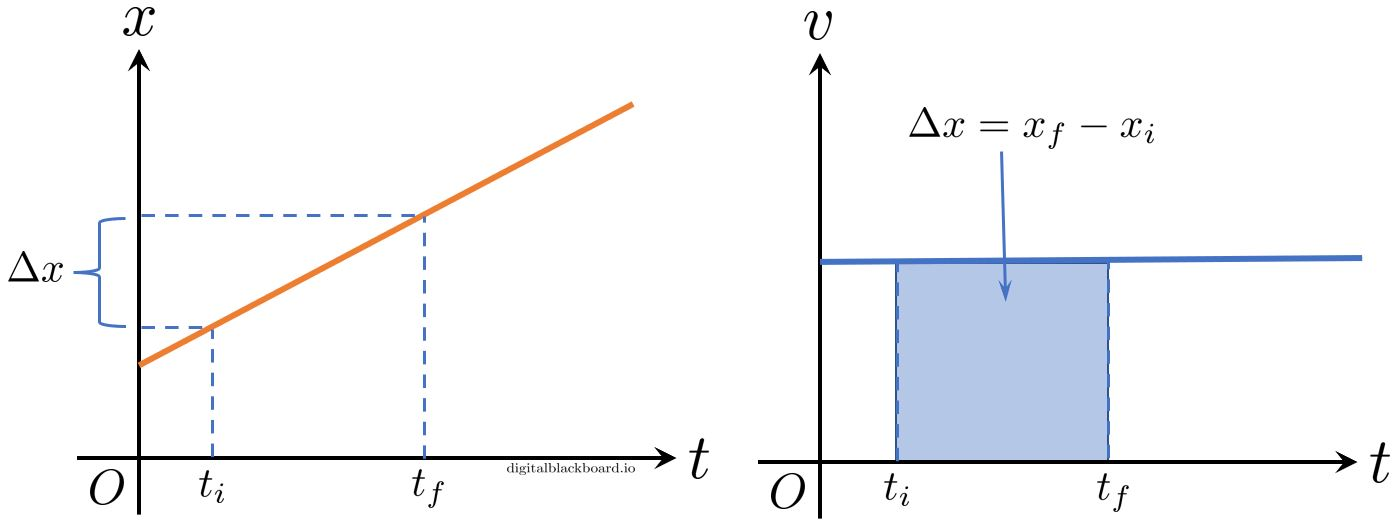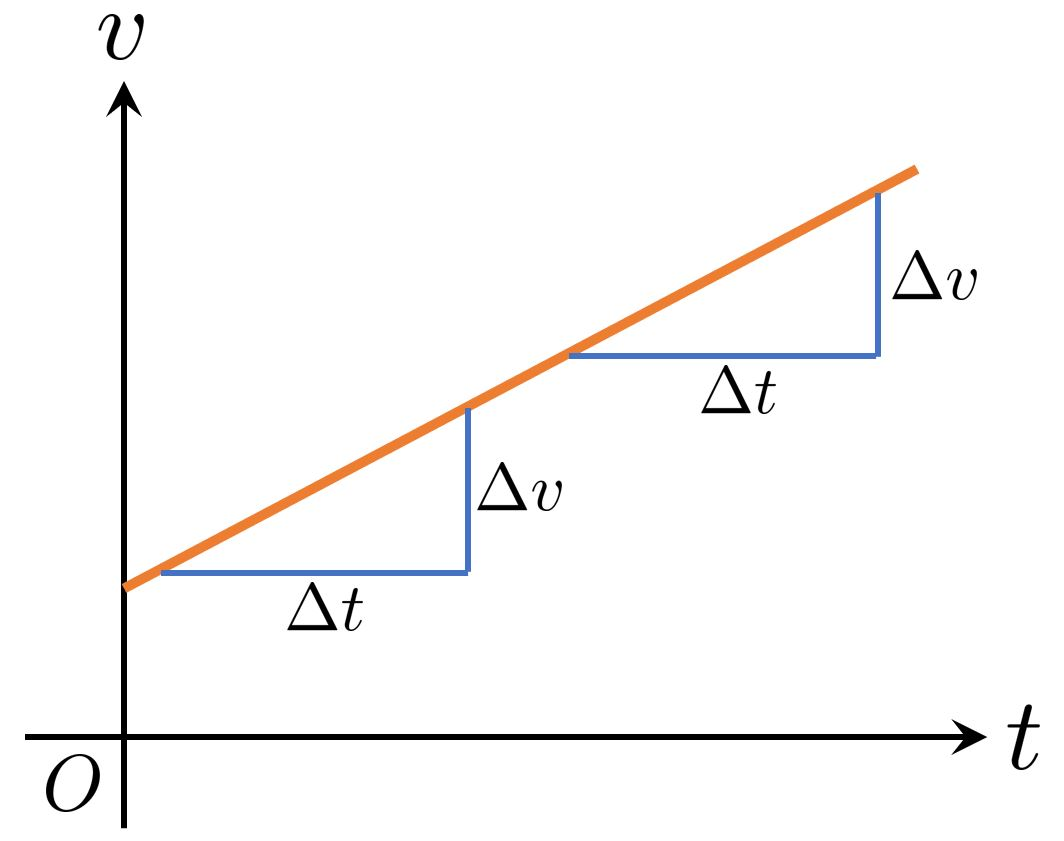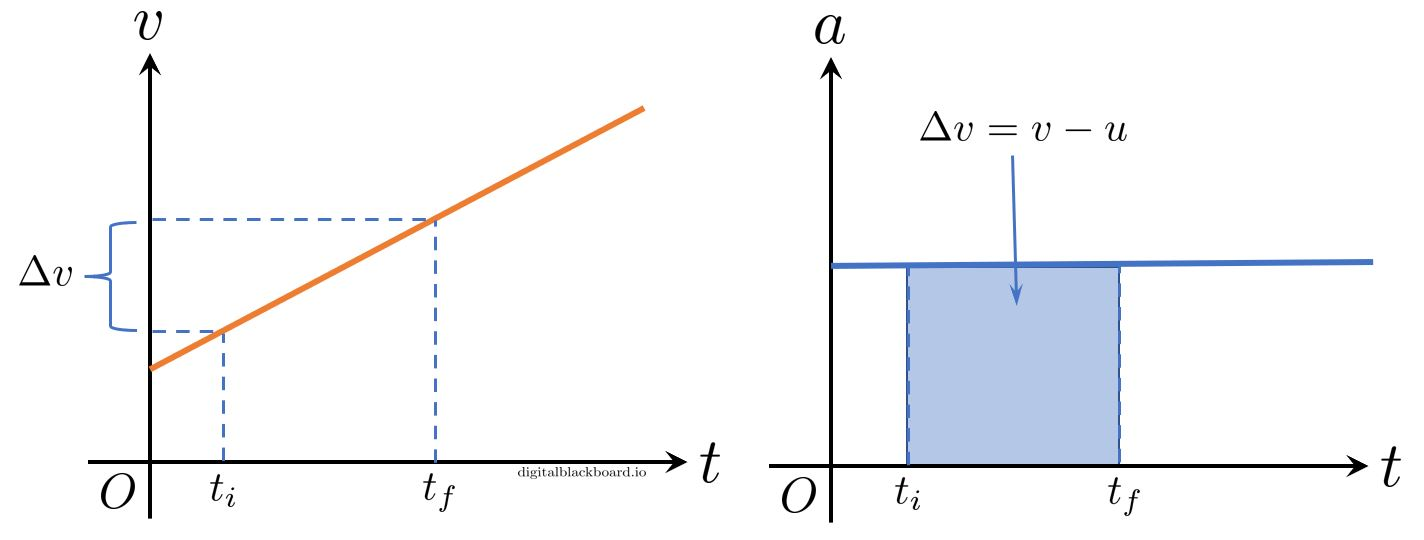## Introduction

• In the previous section, we defined the kinematic quantities used in the study of kinematics, including displacement, velocity and acceleration and the relationships between these quantities.

• In this section, we derive the kinematic equations of motion and demonstrate their applications for two special cases:

• uniform motion
• uniformly accelerated motion.

## Uniform Motion

• By uniform motion, we mean a motion where the velocity is constant. In other words, the $x$-$t$ graph is a straight line with a constant slope. In equal intervals of time $\Delta t$, the position changes by the same amount $\Delta x$.### Equations of Motion

• We recall the definition of velocity,

$$v(t)=\frac{dx(t)}{dt}.$$

• We now express $dx(t)$ as the subject and integrate both sides:

$$\int_{x_0}^{x_f} dx(t) = \int_{t_i}^{t_f} v(t)dt.$$

where $x_0$ and $x_f$ are the initial and final positions corresponding to the initial and final times $t_i$ and $t_f$, respectively.

• Since we are dealing with uniform motion, $v(t) = \overline{v}$ is a constant and can be taken out of the integral. Furthermore, we start our clock when motion starts: $t_i=0$. Finally, we let the final time and position be $t_f = t$ and $x_f = x$, respectively.

$$\int_{x_0}^{x} dx(t) = \overline{v} \int_{0}^{t} dt,$$

$$x - x_0 = \overline{v}t,$$

 $$\boxed{x = x_0 + \overline{v}t}$$ (1)
• The above equation allows us to compute the final position $x$ of an object given its initial position $x_0$, constant velocity $\overline{v}$ and the elapsed time $t$.

### Graphical Methods

• The definition of velocity as the rate of change of position tells us that the slope of the $x$-$t$ graph is the velocity.

• Also, we deduce that the area under the $v$-$t$ graph is the displacement:

$$\int_{x_0}^{x_f} dx(t) = \int_{t_i}^{t_f} v(t)dt.$$

$$x_f-x_0 = \int_{t_i}^{t_f} v(t)dt.$$EXAMPLE : Solving uniform motion problems using both equations and graphs.

Two runners in a marathon, $A$ and $B$, start running at the same time. The initial position of $A$ is $0~$m and his velocity is $+20~$km/h. The initial position of $B$ is $150~$km and his velocity is $-30~$km/h. Determine the time and position at which they meet

(a) using the kinematic equations.
(b) using a graphical method.

Solution (click to expand)

EXAMPLE : Solving uniform motion problems using both equations and graphs.

Two balls start out moving to the right with constant velocities of $5.0~$m/s and $4.0~$m/s. The slow ball starts first and the other $4.0~$s later. Determine the position of the balls when they meet

(a) using the kinematic equations.
(b) using a graphical method.

Solution (click to expand)
$t=20~$s.

## Uniformly Accelerated Motion

• In a uniformly accelerated motion, the object moves with a constant acceleration. In other words, the $v$-$t$ graph is a straight line with a constant slope. In equal intervals of time $\Delta t$, the velocity changes by the same amount $\Delta v$.### Equations of Motion

• We recall the definition of acceleration,

$$a(t)=\frac{dv(t)}{dt}.$$

• We now express $dx(t)$ as the subject and integrate both sides:

$$\int_{u}^{v} dv(t) = \int_{t_i}^{t_f} a(t)dt.$$

where $u$ and $v$ are the initial and final velocities corresponding to the initial and final times $t_i$ and $t_f$, respectively.

• Since we are dealing with uniformly-accelerated motion, $a(t) = \overline{a}$ is a constant and can be taken out of the integral. Furthermore, we start our clock when motion starts: i.e. $t_i=0$ and let the final time be $t_f = t$.

$$\int_{u}^{v} dv(t) = \overline{a} \int_{0}^{t} dt,$$

$$v - u = \overline{a}t,$$

 $$\boxed{v = u + \overline{a}t}$$ (2)
• The above equation allows us to compute the final velocity $v$ of an object given its initial velocity $u$, constant acceleration $\overline{a}$ and the elapsed time $t$.

• Further, we now invoke the definition of the velocity,

$$v(t)=\frac{dx(t)}{dt},$$

substitute equation (2) and integrate both sides to obtain:

$$\int_{t_0}^t (u + \overline{a}t )dt = \int_{x_0}^x dx(t)$$

$$\left[ ut + \overline{a}\frac{t^2}{2} \right]_{t_0}^t = x - x_0.$$

Finally, assuming we start our clock when motion starts (i.e. $t_0=0$):

 $$\boxed{x = x_0 + ut + \frac{1}{2}\overline{a}t^2}$$ (3)

which allows us to compute the final position $x$ of an object given its initial position $x_0$, initial velocity $u$, constant acceleration $\overline{a}$ and the elapsed time $t$ (in a uniformly-accelerated motion).

• Now, let us derive another relationship by eliminating the time $t$ from equations (2) and (3). We first express $t$ as the subject in equation (2):

$$t = \frac{v-u}{\overline{a}}$$

which is then substituted into equation (3) to obtain

$$x = x_0 + u\left(\frac{v-u}{\overline{a}}\right) + \frac{1}{2}\overline{a}\left(\frac{v-u}{\overline{a}}\right)^2$$

Simplifying and rearranging, we obtain:

 $$\boxed{v^2 = u^2 + 2 \overline{a} (x - x_0)}$$ (4)
• Equations (2), (3) and (4) will be used to solve uniformly-accelerated motion problems.

### Graphical Methods

• The definition of acceleration as the rate of change of velocity tells us that the slope of the $v$-$t$ graph is the acceleration.

• Also, we deduce that the area under the $a$-$t$ graph is the change in velocity $\Delta v$:

$$\int_{u}^{v} dv(t) = \int_{t_i}^{t_f} a(t)dt.$$

$$\Delta v = v-u = \int_{t_i}^{t_f} a(t)dt.$$EXAMPLE : Calculating distance travelled from acceleration and elapsed time.

Suppose a car accelerates from rest at a constant acceleration of $26.0~$m/s$^2$ for $5.56~$s. How far does it travel in this time? Solve the question with the help of a $v$-$t$ graph.

Solution (click to expand)

x=402 m.

EXAMPLE : Solving problems with uniform acceleration.

A car in rectilinear motion has an initial velocity of $3.0~$m/s at time $t=0~$s and accelerates uniformly at $2.0~$m/s$^2$. At $t=10~$s,

(a) what is the velocity of the car?
(b) what is the displacement of the car?

Solution (click to expand)

EXAMPLE : Solving problems with uniform acceleration.

An airplane lands with an initial velocity of $70.0~$m/s and then accelerates opposite to the motion at $1.50~$m/s$^2$ for $40.0~$s. What is its final velocity?

Solution (click to expand)
Ans. $10.0~$m/s.

EXAMPLE : Solving problems with uniform acceleration.

Two cars $A$ and $B$ are travelling in the same direction. $A$ and $B$ are travelling at constant speeds of $40~$m/s and $25~$m/s, respectively. At time $t=0~$s, $A$ overtakes $B$ which immediately accelerates uniformly for $20~$s to reach a constant speed of $50~$m/s. Calculate

(a) the distance car $A$ travels in the first $20~$s.
(b) the acceleration of car $B$ in the first $20~$s.
(c) the distance car $B$ travels in the first $20~$s.
(d) the additional time (beyond $t=20.0~$s) for car $B$ to catch up with car $A$.
(e) the distance each car has travelled when they meet.
(f) the maximum distance between the cars before car $B$ catches up with car $A$.

Solution (click to expand)

EXAMPLE : Solving problems with uniform acceleration.

On dry concrete, a car can accelerate opposite to the motion at a rate of $7.00~$m/s$^2$, whereas on wet concrete it can accelerate opposite to the motion at only $5.00~$m/s$^2$. Find the distances necessary to stop a car moving at $30.0~$m/s (about $110~$km/h) on

(a) dry concrete and
(b) wet concrete.
(c) Repeat both calculations and find the displacement from the point where the driver sees a traffic light turn red, taking into account his reaction time of $0.500~$s to get his foot on the brake.

Solution (click to expand)
(a)x=64.3m on dry concrete.
(b)x=90.0m on wet concrete.
Repeat
(a) to be 64.3 m + 15.0 m = 79.3 m
(b) to be 90.0 m + 15.0 m = 105 m when wet.